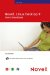< Day Day Up >

Calc is designed to do calculations. Although many built-in formulas and functions are provided by Calc, in some situations you will need to build your own formulas. These formulas typically involve simple math operations such as subtraction, multiplication, and division.

All formulas that you create must begin with the equal sign (=). This tells Calc that the information that follows the equal sign is a formula. To designate what type of calculation you want Calc to perform you use a mathematical operator (see Table 12.1).

##### Table 12.1. Mathematical Operators

OPERATOR

PERFORMS

EXAMPLE

+

=A1+B1

_

Subtraction

=A1-B1

*

Multiplication

=A1*B1

/

Division

=A1/B1

^

Exponentiation

=A1^2

You enter the cell addresses for the cells that will be acted on by the formula. For example, B5*C5 multiplies the contents of B5 times the contents of C5.

When you design your own formulas, keep the natural order of math operator precedence in mind: Exponents are computed first, then multiplication and division, followed by addition and subtraction. To force the precedence you can place portions of your formula in parentheses. This part of the formula is computed first.

 < Day Day Up >Novell Linux Desktop 9 Users Handbook
ISBN: 0672327295
EAN: 2147483647
Year: 2003
Pages: 244
Authors: Joe Habraken